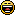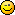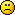Author Topic: Vector 2D  (Read 5259 times)Dark SchneiderVector 2D
« on: 2011-Jan-18 »
Typical case of 2D-Vector, very usefull for sprites and other 2D things:

Code: GLBasic [Select]
// --------------------------------- //
// TYPE TVector2
// --------------------------------- //

CONSTANT TVector2VersionMajor%=1, TVector2VersionMinor%=1, TVector2VersionBuild%=0, TVector2VersionRevision%=2

//! TVector2
//!
//! Author: Dark Schneider
//!
//! Two-dimensional vector

//---------------------//
// --- DEFINITIONS --- //
//---------------------//

//---------------//
// --- TYPES --- //
//---------------//
//! TVector2 types

//! Type TVector2
//! Main type
TYPE TVector2

//---------------//
// - VARIABLES - //
//---------------//
x#=0
y#=0

//---------------//
// - FUNCTIONS - //
//---------------//
//! TVector2 functions

//! Set type data
//\param x# - coordinate X
//\param y# - coordinate Y
//\return Itself with new values
FUNCTION Set AS TVector2: x#=0, y#=0
self.x=x; self.y=y
RETURN self
ENDFUNCTION

//\return Length squared of vector
FUNCTION LengthSq:
RETURN self.x*self.x+self.y*self.y
ENDFUNCTION

//\return Length of vector
FUNCTION Length:
RETURN SQR(self.x*self.x+self.y*self.y)
ENDFUNCTION

//\param v - vector for dot product
//\return Dot product of itself with v
FUNCTION DotProduct: v AS TVector2
RETURN self.x*v.x+self.y*v.y
ENDFUNCTION

//\param scale# - scale factor
//\return Itself scaled
FUNCTION Scale AS TVector2: scale#
self.x=self.x*scale#
self.y=self.y*scale#
RETURN self
ENDFUNCTION

//\return Itself normalized
FUNCTION Normalize AS TVector2:
LOCAL l#=self.Length()
IF l < 0.0000001
self.Set(0,0)
ELSE
self.Scale(1.0/l)
ENDIF
RETURN self
ENDFUNCTION

//\return Itself with inverse values: x=-x; y=-y
FUNCTION Invert AS TVector2:
self.x=-self.x
self.y=-self.y
RETURN self
ENDFUNCTION

//\return New vector with inverse values: x=-x; y=-y
FUNCTION Inverse AS TVector2:
LOCAL l_result AS TVector2
l_result=self
l_result.Invert()
RETURN l_result
ENDFUNCTION

//\param v - vector to add
//\return Itself modified
FUNCTION Add AS TVector2: v AS TVector2
self.x=self.x+v.x
self.y=self.y+v.y
RETURN self
ENDFUNCTION

//\param v - vector to substract
//\return Itself modified
FUNCTION Substract AS TVector2: v AS TVector2
self.x=self.x-v.x
self.y=self.y-v.y
RETURN self
ENDFUNCTION

//! Compute angle between the vector and another one
//\param v - vector against to compute angle
//\return Angle from itself to v, values in the range [0-180[
FUNCTION AngleWith: v AS TVector2
LOCAL div#, dot#
div = self.Length() * v.Length()
IF div = 0 THEN RETURN 0
dot = self.DotProduct(v) / div
IF ABS(dot) > 1 THEN RETURN 0 // 1.5/1.49999
RETURN ACOS(dot)
ENDFUNCTION

//\return Angle of the vector using X axis as angle=0
FUNCTION ToAngle:
LOCAL l_result#=0
IF self.x<>0 AND self.y<>0
l_result=ATAN(self.y,self.x)
IF self.y<0 THEN l_result=360+l_result
ELSE
IF self.x=0
IF self.y>0
l_result=90
ELSEIF self.y<0
l_result=270
ENDIF
ELSEIF self.y=0 AND self.x<0
l_result=180
ENDIF
ENDIF
RETURN l_result
ENDFUNCTION

//! Modify the vector creating a normalized vector with direction defined by an angle
//\return Itself modified
FUNCTION FromAngle AS TVector2: angle#
self.x=COS(angle)
self.y=SIN(angle)
RETURN self
ENDFUNCTION

ENDTYPE

//-------------------//
// --- FUNCTIONS --- //
//-------------------//
//! Global functions

//! Create a new vector instance
//\param x# - coordinate X
//\param y# - coordinate Y
//\return The new vector instance
FUNCTION TVector2Create AS TVector2: x#=0, y#=0
LOCAL l_result AS TVector2
l_result.Set(x,y)
RETURN l_result
ENDFUNCTION

//! Add 2 vectors
//\param v1 - vector1
//\param v2 - vector2
//\return New vector v=v1+v2
FUNCTION TVector2Add AS TVector2: v1 AS TVector2, v2 AS TVector2
LOCAL l_result AS TVector2
l_result=v1
RETURN l_result
ENDFUNCTION

//! Substract 2 vectors
//\param v1 - vector1
//\param v2 - vector2
//\return New vector v=v1-v2
FUNCTION TVector2Substract AS TVector2: v1 AS TVector2, v2 AS TVector2
LOCAL l_result AS TVector2
l_result=v1
l_result.Substract(v2)
RETURN l_result
ENDFUNCTION

//! Scale a vector
//\param v - vector to use as base for scale
//\scale# - scale factor
//\return New vector v*scale
FUNCTION TVector2Scale AS TVector2: v AS TVector2, scale#
LOCAL l_result AS TVector2
l_result=v
l_result.Scale(scale#)
RETURN l_result
ENDFUNCTION

//! Compute a normalized vector with direction defined by an angle
//\return New vector
FUNCTION TVector2FromAngle AS TVector2: angle#
LOCAL l_result AS TVector2
l_result.FromAngle(angle)
RETURN l_result
ENDFUNCTION

//! Computes the linear interpolation between 2 vectors
//\param v1 - vector1
//\param v2 - vector2
//\param interpolation# - interpolation value, [0.0-1.0]
//\return Vector with values of the interpolation
FUNCTION TVector2LinearInterpolation AS TVector2: v1 AS TVector2, v2 AS TVector2, interpolation#
LOCAL l_vresult AS TVector2
l_vresult.x=(1.0-interpolation)*v1.x+interpolation*v2.x
l_vresult.y=(1.0-interpolation)*v1.y+interpolation*v2.y
RETURN l_vresult
ENDFUNCTIONMoru

• Prof. Inline
••• Posts: 1775Re: Vector 2D
« Reply #1 on: 2011-Jan-18 »
Very nice, saving for later exploration :-)Schranz0r

• Premium User :)
• Prof. Inline
••• Posts: 5022
• O Rly?Re: Vector 2D
« Reply #2 on: 2011-Jan-18 »
I like that!
IDGArray'sPC:
AMD Ryzen 7 1700 @3.9GHz, 16GB HyperX Fury 3000MHz Ram, ASUS ROG GTX 1060 STRIX 6GB, Windows 10 Pro 64Bit, MSi Tomahawk B350 MainboardzxeviousRe: Vector 2D
« Reply #3 on: 2012-Mar-07 »
Very, very useful for IAThank you very much indeed!
Best Regards.

Mass Effect 2 Fan!erico

• Community Developer
• Prof. Inline
••• Posts: 4218Re: Vector 2D
« Reply #4 on: 2012-Mar-07 »
This seems to be great!
...but beyond my capabilities on understanding...spicypixel

• Prof. Inline
••• Posts: 646
• Pixel ArtistRe: Vector 2D
« Reply #5 on: 2012-Mar-08 »
This seems to be great!
...but beyond my capabilities on understanding...I was wondering what it was tooComps Owned - ZX.81, ZX.48K, ZX.128K+2, Vic20, C64, Atari-ST, A500.600.1200, PC, Apple Mini-Mac.bigsofty

• Community Developer
• Prof. Inline
••• Posts: 2628Re: Vector 2D
« Reply #6 on: 2012-Mar-08 »
Not keen on how this has been done, here's an example.

Code: GLBasic [Select]
a as TVector2
b as TVector2
a.x=100
a.y=100
b.x=10
b.y=20
//add 2 vectors a and b

This is a way of adding to vectors BUT what if you wanted to store the result in another vector and not change 'a'? You cant because the 'add' and most other functions actually modify the types own values each time you call a function. This will lead to unexpected results.

A better way would be to simply return a modified temporary type like.

Code: GLBasic [Select]
//\param v - vector to add
//\return Itself modified
FUNCTION Add AS TVector2: v AS TVector2
self.x=self.x+v.x
self.y=self.y+v.y
RETURN self
ENDFUNCTION

becomes...

Code: GLBasic [Select]
//\param v - vector to add
//\return Itself modified
FUNCTION Add AS TVector2: v AS TVector2
local tmpV as TVector2
tmpV.x=self.x+v.x
tmpV.y=self.y+v.y
RETURN tmpV
ENDFUNCTION

Then this is possible...

Code: GLBasic [Select]
a as TVector2
b as TVector2
c as TVector2
a.x=100
a.y=100
b.x=10
b.y=20
//add 2 vectors a and b

or even...

P.S. none of this has been compiled and I haven't used this code so be aware of typos!